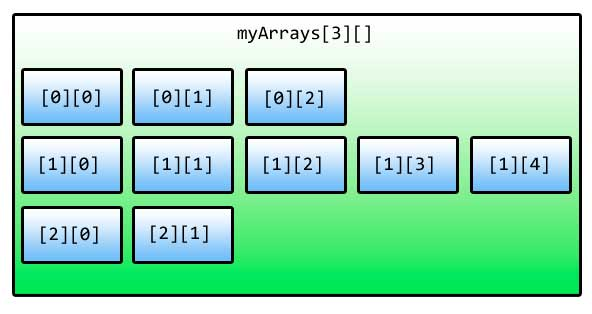Jagged Arrays are multidimensional arrays containing rows of varying lengths. A simple multidimensional array is rectangular because it’s rows has the same number of columns, but jagged arrays can have different lengths for each of it’s rows. Therefore, jagged arrays can also be called arrays of arrays. Below shows the syntax of declaring a jagged array.

``datatype[][] arrayName;``

We write the data type followed by two sets of square brackets to indicate that it is a jagged array. Initializing jagged arrays with values is quite confusing. The following is an example of one way of initializing a jagged array.

``````int[][] myArrays = new int[];

myArrays = new int;
myArrays = new int;
myArrays = new int;``````

First, we declared the number of “rows” of the jagged array by using the new keyword, the data type and two pairs of square brackets with the number of rows inside the first pair. Then we give the number of columns for each row by accessing those three rows by their indices and initializing them like normal arrays. You can give each row a set of values for their columns.

``````int[][] myArrays = new int[];

myArrays = new int { 1, 2, 3 };
myArrays = new int { 5, 4, 3, 2, 1 };
myArrays = new int { 11, 22 };``````

Another clever way of initializing a jagged array is as follows.

``````int[][] myArrays = new int[] { new int { 1, 2, 3 },
new int { 5, 4, 3, 2, 1 },
new int { 11, 22 } };``````

Also, you can ignore specifying the lengths of each row by simply not indicating them.

``````int[][] myArrays = new int[][] { new int[] { 1, 2, 3 },
new int[] { 5, 4, 3, 2, 1 },
new int[] { 11, 22 } };``````

You can simplify the above code even more.

``````int[][] myArrays = { new int[] { 1, 2, 3 },
new int[] { 5, 4, 3, 2, 1 },
new int[] { 11, 22 } };``````

We access a value of an element of a jagged array by indicating its row and column indices.

``array[row][column]``
``Console.WriteLine(myArrays);``

We can’t use a single foreach loop to access the each element of a jagged array.

``````foreach(int array in myArrays)
{
Console.WriteLine(array);
}``````

This will produce an error because what we are extracting in the myArrays are arrays as well. So to fix this, we have to change the type of the temp variable into an int array and nest another foreach loop to access the values of that temp array variable.

``````foreach(int[] array in myArrays)
{
foreach(int number in array)
{
Console.WriteLine(number);
}
}``````

Alternatively, you can use a nested for loop.

``````for (int row = 0; row < myArray.Length; row++)
{
for (int col = 0; col < myArray[row].Length; col++)
{
Console.WriteLine(myArray[row][col]);
}
}``````

On the first for loop, we access the Length property of myArray to get the number of rows of the jagged array. On the second for loop, we use the Length property of the current row element to get the number of columns. We then print the array element with indices indicated by the current value of row and col.